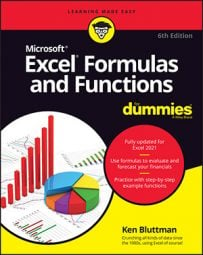##### Excel Formulas & Functions For DummiesIn Excel formulas, you can refer to other cells either relatively or absolutely. When you copy and paste a formula in Excel, how you create the references within the formula tells Excel what to change in the formula it pastes. The formula can either change the references relative to the cell where you're pasting it (relative reference), or it can always refer to a specific cell.

You can also mix relative and absolute references so that, when you move or copy a formula, the row changes but the column does not, or vice versa.

Preceding the row and/or column designators with a dollar sign (\$) specifies an absolute reference in Excel.

Example Comment
=A1 Complete relative reference
=\$A1 The column is absolute; the row is relative
=A\$1 The column is relative; the row is absolute
=\$A\$1 Complete absolute reference